Courses

# Influence Lines for Beams - 4 Civil Engineering (CE) Notes | EduRev

## Civil Engineering (CE) : Influence Lines for Beams - 4 Civil Engineering (CE) Notes | EduRev

The document Influence Lines for Beams - 4 Civil Engineering (CE) Notes | EduRev is a part of the Civil Engineering (CE) Course Structural Analysis.
All you need of Civil Engineering (CE) at this link: Civil Engineering (CE)

Absolute maximum moment in s beam supporting a series of moving concentrated loads.

In earlier sections, we have learned to compute the maximum shear and moment for single load, UDL and series of concentrated loads at specified locations. However, from design point of view it is necessary to know the critical location of the point in the beam and the position of the loading on the beam to find maximum shear and moment induced by the loads. Following paragraph explains briefly for the cantilever beam or simply supported beam so that quickly maximum shear and moment can be obtained.

Maximum Shear: As shown in the Figure 39.22, for the cantilever beam, absolute maximum shear will occur at a point located very near to fixed end of the beam. After placing the load as close as to fixed support, find the shear at the section close to fixed end.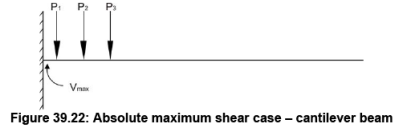Similarly for the simply supported beam, as shown in Figure 39.23, the absolute maximum shear will occur when one of the loads is placed very close to support.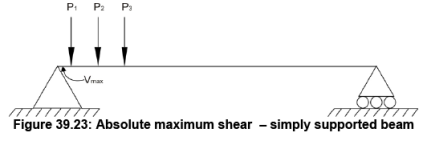Moment:
The absolute maximum bending moment in case of cantilever beam will occur where the maximum shear has occurred, but the loading position will be at the free end as shown in Figure 39.24.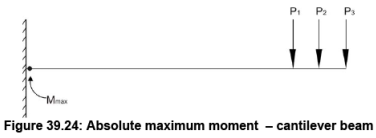The absolute maximum bending moment in the case of simply supported beam, one cannot obtain by direct inspection. However, we can identify position analytically. In this regard, we need to prove an important proposition.

Proposition:
When a series of wheel loads crosses a beam, simply supported ends, the maximum bending moment under any given wheel occurs when its axis and the center of gravity of the load system on span are equidistant from the center of the span.

Let us assume that load P1, P2, P3 etc. are spaced shown in Figure 39.25 and traveling from left to right. Assume PR to be resultant of the loads, which are on the beam, located in such way that it nearer to P3 at a distance of d1 as shown in Figure 39.25.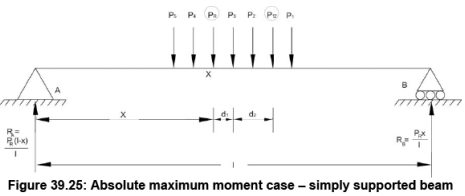If P12 is resultant of P1 and P2, and distance from P3 is d2. Our objective is to find the maximum bending moment under load P3. The bending moment under P3 is expressed as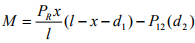Differentiate the above expression with respect to x for finding out maximum moment.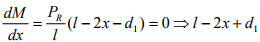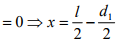Above expression proves the proposition.

Let us have a look to some examples for better understanding of the abovederived proposition.

Numerical Examples

Example 1:
The beam is loaded with two loads 25 kN each spaced at 2.5 m is traveling on the beam having span of 10 m. Find the absolute maximum moment

Solution:
When the a load of 25kN and center of gravity of loads are equidistant from the center of span then absolute bending moment will occur. Hence, place the load on the beam as shown in Figure 39.26.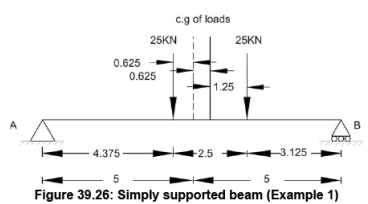The influence line for Mx is shown in Figure 39.27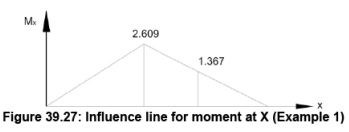Computation of absolute maximum moment is given below.

Mx = 25(2.461) + 25(1.367) = 95. 70 kN.m

Example 2:
Compute the absolute maximum bending moment for the beam having span of 30 m and loaded with a series of concentrated loads moving across the span as shown in Figure 39.28.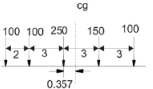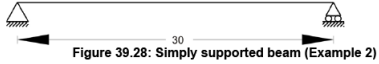First of all compute the center of gravity of loads from first point load of 100 kN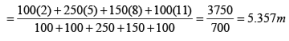Now place the loads as shown in Figure 39.29.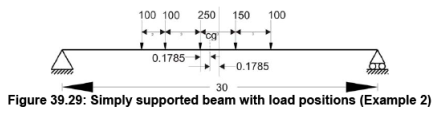Also, draw the influence line as shown in Figure 39.30 for the section X.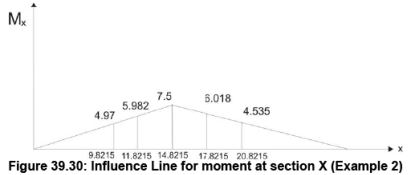Mx = 100(4.97) + 100(5.982) + 250(7.5) + 150(6.018) + 100(4.535) = 4326.4kN.m

Envelopes of maximum influence line values

For easy calculations steps of absolute maximum shear and moment rules for cantilever beam and simply supported beam were discussed in previous section. Nevertheless, it is difficult to formulate such rules for other situations. In such situations, the simple approach is that develop the influence lines for shear and moment at different points along the entire length of the beam. The values easily can be obtained using the concepts developed in earlier sections. After obtaining the values, plot the influence lines for each point under consideration in one plot and the outcome will be envelop of maximums. From this diagram, both the absolute maximum value of shear and moment and location can be obtained. However, the approach is simple but demands tedious calculations for each point. In that case, these calculations easily can be done using computers.

Offer running on EduRev: Apply code STAYHOME200 to get INR 200 off on our premium plan EduRev Infinity!

## Structural Analysis

30 videos|122 docs|28 tests

,

,

,

,

,

,

,

,

,

,

,

,

,

,

,

,

,

,

,

,

,

;